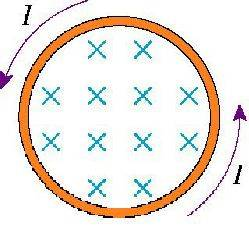# Simple Magnetic Field Increase/Decrease question.

• Metalsonic75

#### Metalsonic75

33.6 There is a ccw-induced current in the conducting loop shown in the figure. Is the magnetic field inside the loop increasing in strength, decreasing in strength, or steady?I don’t understand if the problem is referring to the induced magnetic field or the pre-existing magnetic field. I know that the induced field will increase, but I’m pretty sure the original electric field remains steady. Which one is the problem asking for?

The problem is asking if the applied magnetic field, the field represented by the X's, is increasing or decreasing. You'll need to use Lenz's Law and the right hand rule for current and magnetic fields to solve this. Does that help?

So, the x's in the picture represent the original magnetic field, right? I assume that means the induced magnetic field (which should point out of the page) is not shown.

Lenz's law says that the induced magnetic field changes in response to an induced current. I don't understand how the induced magnetic field and the original magnetic field are related.

My textbook says that for a loop with a counterclockwise current and an applied magnetic field pointing into the page, the induced magnetic field will point out of the page, and it says that the "induced field is increasing." I am not seeing any information regarding how the applied field is affected by the introduction of a current, leading me to believe it is not affected and does not change.

Ok, you've made some good observations but let's be very clear about what's going on here. There is a current I in the wire loop. This is an induced current caused by the applied magnetic field. The applied magnetic field changes with respect to time (we know this by Faraday's Law). We want to know if this magnetic field is changing positively or negatively, that is, is $$\Delta B$$ positive or negative.

Lenz's Law tells us that the induced magnetic field, that is, the magnetic field caused by the induced current, will act to oppose the change in the applied magnetic field. You've already mentioned that the induced magnetic field points out of the page inside the loop, so which direction must the change in the applied magnetic field point? And subsequently, does this constitute an increase or decrease in the applied field?

Last edited:
Thank you very much. I now understand the concepts of Lenz's law and magnetic fields much better, and I was able to obtain the correct answer. :)